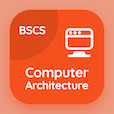Computer Science Courses Online

Database Management System Practice Tests

Database Management System Online Tests

The Book Domain Relational Calculus Multiple Choice Questions (MCQ Quiz) with answers, Domain Relational Calculus MCQ Quiz PDF download to study online database management system degree courses. Practice Relational Algebra and Calculus Multiple Choice Questions and Answers (MCQs), Domain Relational Calculus quiz answers PDF for CS major. The e-Book Domain Relational Calculus MCQ App Download: division operation, tuple relational calculus, binary relational operation: join and division, select and project test prep for best online schools for computer science.

The MCQ: The branch of calculus which is based on mathematical logic is classified as PDF, "Domain Relational Calculus" App Download (Free) with universal calculus, predicate calculus, indicate calculus, and local calculus choices for CS major. Study domain relational calculus quiz questions, download Google eBook (Free Sample) for applied computer science.

## Database Management System MCQs: Domain Relational Calculus Quiz Questions

MCQ: The branch of calculus which is based on mathematical logic is classified as

A) universal calculus
B) predicate calculus
C) indicate calculus
D) local calculus

MCQ: In the relational calculus, the variables are bounded with the help of

A) non-identifiers
B) non-quantifiers
C) quantifiers
D) identifiers

MCQ: The types of relational calculi includes

A) domain calculus
B) nodes calculus
C) tuple calculus
D) both a and c

MCQ: The relational calculus is classified as

A) high level language
B) low level language
C) query specifying language
D) query identifying language

### Practice Tests: Database Management System Exam Prep

Download Database Management System Quiz App, Computer Architecture MCQ App, and Digital Logic Design MCQs App to install for Android & iOS devices. These Apps include complete analytics of real time attempts with interactive assessments. Download Play Store & App Store Apps & Enjoy 100% functionality with subscriptions!